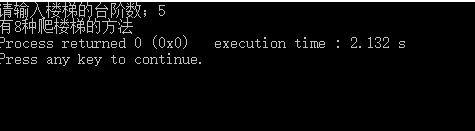# 第12周OJ实践 爬楼梯

/*烟台大学计算机学院

*/
#include <stdio.h>
#include <stdlib.h>
unsigned long count (int n);
int main()
{

int n;
unsigned long m;
printf("请输入楼梯的台阶数；");
scanf("%d",&n);
m=count(n);//构造中间变量
printf("有%lu种爬楼梯的方法",m);
return 0;
}
unsigned long count (int n)
{
unsigned long f;
if(n==1)
f=1;
else if(n==2)
f=2;
else
f=count(n-1)+count(n-2);
return f;
}两个台阶，有2种走法；
有n个台阶时，设有count(n)种走法，最后一步走1个台阶，有count(n-1)种走法；最后一步走2个台阶，有count(n-2)种走法。于是count(n)=count(n-1)+count(n-2)。

unsigned long int   无符号型长整形，数据全为正数，范围较大。